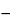Ascorbic Acid
(as kore' bik as' id).

C6H8O6 176.12
l-Ascorbic acid[50-81-7].
DEFINITION
Ascorbic Acid contains NLT 99.0% and NMT 100.5% of C6H8O6.
IDENTIFICATION
•  B. A 20-mg/mL solution reduces alkaline cupric tartrate TS slowly at room temperature but more readily upon heating.
ASSAY
•  Procedure
Sample:  400 mg of Ascorbic Acid
Titrimetric system
Mode:  Direct titration
Titrant:  0.1 N iodine VS
Endpoint detection:  Visual
Blank:  100 mL of water and 25 mL of 2 N sulfuric acid. Add 3 mL of starch TS.
Analysis:  Dissolve the Sample in a mixture of 100 mL of water and 25 mL of 2 N sulfuric acid. Add 3 mL of starch TS, and titrate immediately with Titrant until a persistent violet-blue color is obtained.
Calculate the percentage of ascorbic acid (C6H8O6) in the portion of Ascorbic Acid taken:
Result = [(VB) × N × F × 100]/W
 V = = sample titrant volume (mL) B = = blank titrant volume (mL) N = = titrant normality (mEq/mL) F = = equivalency factor, 88.06 mg/mEq W = = weight of Sample (mg)
Acceptance criteria:  99.0%–100.5%
IMPURITIES
Sample solution:  1 g in 25 mL of water
Acceptance criteria:  NMT 20 ppm
SPECIFIC TESTS
Sample solution:  100 mg/mL in carbon dioxide-free water. Perform the test immediately after preparation of the Sample solution.
Acceptance criteria:  +20.5to +21.5